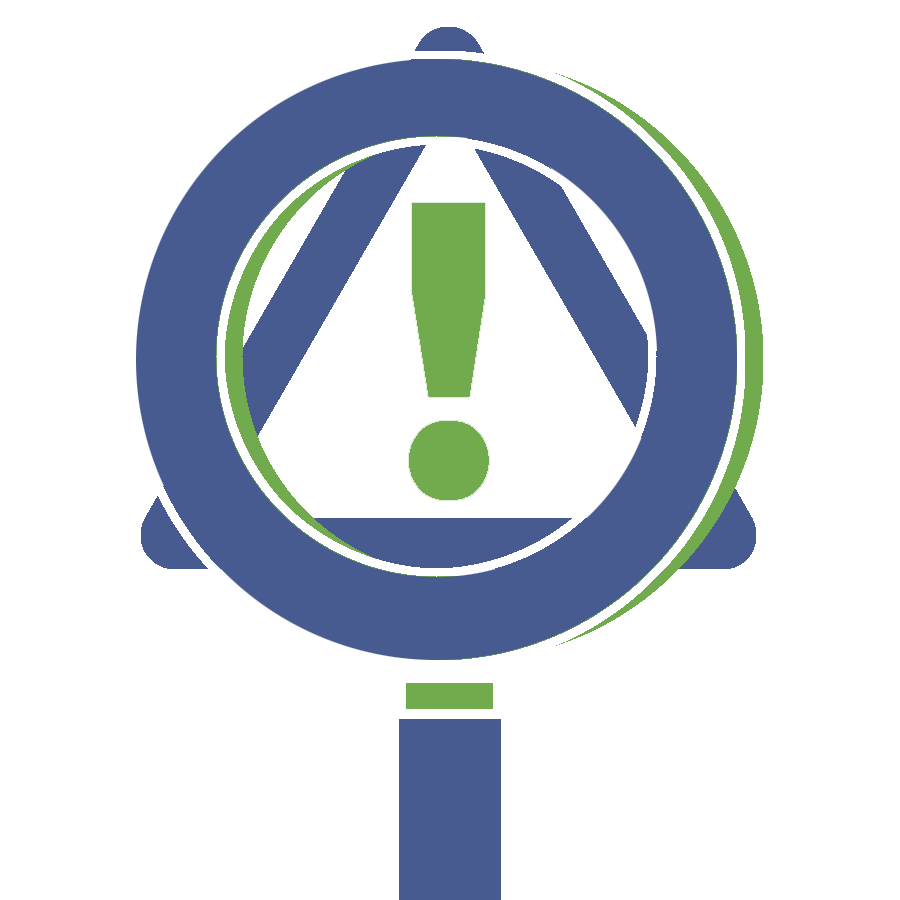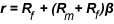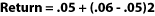## Analysis Intermediate: Evaluating Risk# Risks and Returns

Generally, the higher the volatility of your investment, the greater the expected return. Investors who take risks expect to be rewarded for taking those risks.

### Things To Know

• The higher the volatility, the greater the expected return.
• The CAPM examines the relationship between risk and return.

## How the capital asset pricing model fits in

The capital asset pricing model (CAPM) examines the relationship between risk and return for a given asset. CAPM is often used as a model for pricing risky securities. CAPM uses a formula to determine a security’s maximum expected return for a given level of risk. It assumes that most investors prefer less risk and will demand compensation for the amount of risk they take.

## The CAPM formulaIn this equation, "r" stands for return, Rm stands for market rate of return, Rf stands for risk-free return (the return of a "risk free" investment such as a Treasury bill), and β represents beta.

## An example

Although the formula looks complicated, it’s not. For example, let’s say you want to know the expected return of a stock with a risk-free rate of return of 5 percent and a market rate of return of 6 percent. The stock’s beta is 2. So:You could expect a return of 7 percent on this investment!